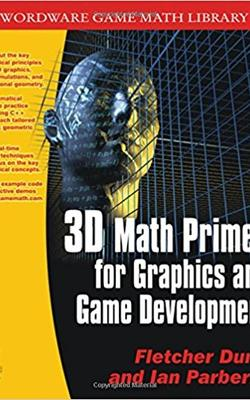# BOOKS AND VIDEOS

### 3D Math Primer for Graphics and Game Development

#### by Fletcher Dunn, Ian Parberry

Published June 21, 2002Mid level Buy Book

3D Math Primer for Graphics and Game Development covers fundamental 3D math concepts that are especially useful for computer game developers and programmers. The authors discuss the mathematical theory in detail and then provide the geometric interpretation necessary to make 3D math intuitive. Working C++ classes illustrate how to put the techniques into practice, and exercises at the end of each chapter help reinforce the concepts. This book explains basic concepts such as vectors, coordinate spaces, matrices, transformations, Euler angles, homogenous coordinates, geometric primitives, intersection tests, and triangle meshes. It discusses orientation in 3D, including thorough coverage of quaternions and a comparison of the advantages and disadvantages of different representation techniques. The text describes working C++ classes for mathematical and geometric entities and several different matrix classes, each tailored to specific geometric tasks. Also included are complete derivations for all the primitive transformation matrices.